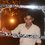# Help needed..

If the $${ r }^{ th }$$ term in the expansion of $${ \left( 2x+\frac { 1 }{ { x }^{ m } } \right) }^{ n },(n\epsilon N)$$ is independent term of $$x$$ and $${ r }^{ th }$$ term in expansion of $${ \left( \frac { 1 }{ { x }^{ 4 } } -2{ x }^{ n-6 } \right) }^{ m },(m\epsilon N)$$ is independent term of $$x$$, then minimum possible value of $$\left[ \frac { { m }^{ 2 }+{ n }^{ 2 } }{ 9 } \right]$$ is? (where $$\left[ . \right]$$ represents greatest integer function)

What I have done is write down the general term in both expansions and equated the powers of $x$ to zero in both cases and tried to connect them...But it goes nowhere..Note by Anandhu Raj
6 years, 1 month ago

This discussion board is a place to discuss our Daily Challenges and the math and science related to those challenges. Explanations are more than just a solution — they should explain the steps and thinking strategies that you used to obtain the solution. Comments should further the discussion of math and science.

When posting on Brilliant:

• Use the emojis to react to an explanation, whether you're congratulating a job well done , or just really confused .
• Ask specific questions about the challenge or the steps in somebody's explanation. Well-posed questions can add a lot to the discussion, but posting "I don't understand!" doesn't help anyone.
• Try to contribute something new to the discussion, whether it is an extension, generalization or other idea related to the challenge.

MarkdownAppears as
*italics* or _italics_ italics
**bold** or __bold__ bold
- bulleted- list
• bulleted
• list
1. numbered2. list
1. numbered
2. list
Note: you must add a full line of space before and after lists for them to show up correctly
paragraph 1paragraph 2

paragraph 1

paragraph 2

[example link](https://brilliant.org)example link
> This is a quote
This is a quote
    # I indented these lines
# 4 spaces, and now they show
# up as a code block.

print "hello world"
# I indented these lines
# 4 spaces, and now they show
# up as a code block.

print "hello world"
MathAppears as
Remember to wrap math in $$ ... $$ or $ ... $ to ensure proper formatting.
2 \times 3 $2 \times 3$
2^{34} $2^{34}$
a_{i-1} $a_{i-1}$
\frac{2}{3} $\frac{2}{3}$
\sqrt{2} $\sqrt{2}$
\sum_{i=1}^3 $\sum_{i=1}^3$
\sin \theta $\sin \theta$
\boxed{123} $\boxed{123}$

Sort by:

- 6 years, 1 month ago

When you try to connect, you got two equations in $m,n \ and \ r$ then substitute the value of $r$ in terms of $m,n$ from one equation in the second equation and simplify.

On simplifying you will get :

$4m^2+4m=n^2-2n$

$4m^2-n^2=-2(2m+n)$

$(2m+n)(2m-n)=-2(2m+n)$

So, either $2m+n=0$ or $2m-n=-2$.

The first one is not possible because $m,n \in \mathbb{N}$ .

So $2m-n=-2$

$2m=n-2$.

Now, we'll focus on the value of $n$ :

From the second equation, it is sure that $n \geq 6$.

If $n=6 , \implies m=2$ (Min. Permissible values)

Hence the answer comes to be $4$.

edited !

Hope you'll enjoy it !

- 6 years, 1 month ago

Why $n > 6?$

$n = 6$, $m = 2$ also satisfies the condition And also gives the minimum value of 4.

Clarification:- check the last term of second equation with n= 6( power of $1/x^4$ equals 0).

- 6 years, 1 month ago

You're right. I made a mistake by neglecting n=6. Thanks !

- 6 years, 1 month ago

Thanks sir :)

- 6 years, 1 month ago